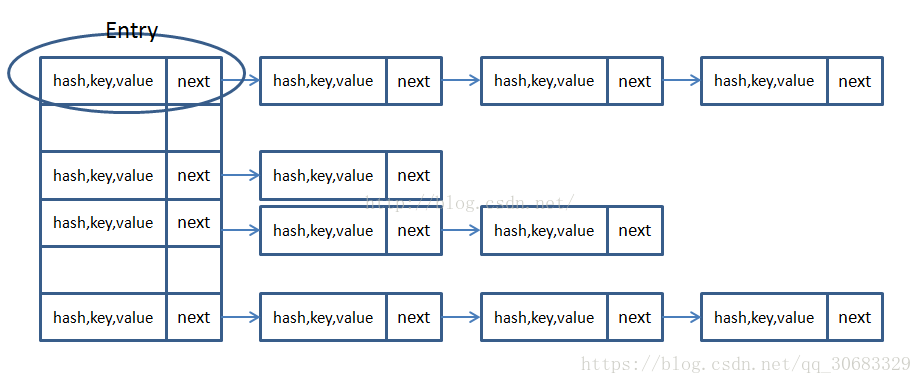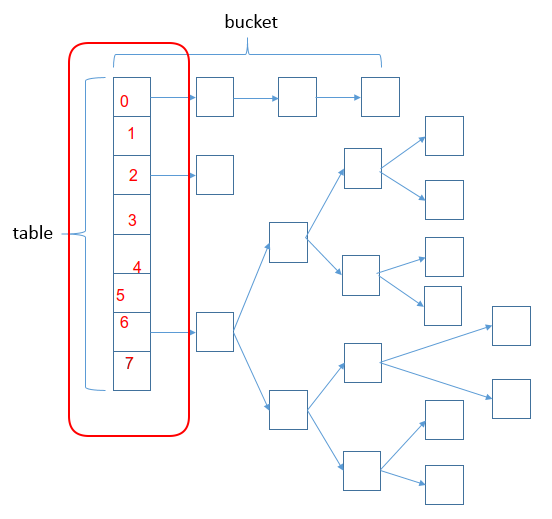Error message here!

Hide Error message here!

Error message here!

Hide Error message here!

Error message here!

Close

# 以最简单的方式讲HashMap

HashMap可以说是面试中最常出现的名词，这次头条的一面，第一个问的问题就是HashMap。所以就让我们来探讨下HashMap吧。

1.HashMap的储存结构1.HashMap中的Hash1.HashMap是怎么保存数据的1.HashMap的扩容操作1.HashMap的线程安全问题

## HashMap的储存结构

### jdk1.7的存储结构### jdk1.8的储存结构## Hash

put(k,v)时，public V put(K key, V value){ return putVal(hash(key), key, value, false, true); }

key算出key的hash值。

• JDK1.8
static final int hash(Object key){ int h; return (key == null) ? 0 : (h = key.hashCode()) ^ (h >>> 16); }

## HashMap保存数据

• initialCapacity：初始化容量默认是16
• capacity：容量，通过initCapacity计算出一个大于或者等于initCapacity且为2的幂的值

1.未产生hash冲突// n是HashMap的大小，Hash为key的hash值，tab为如下图中的table，i代表储存的位置 int i; // 为null代表此位置为空的 if ((p = tab[i = (n - 1) & hash]) == null) tab[i] = newNode(hash, key, value, null);例如：当某一hash值与（n-1）相与的结果是3，那么就将这个这个table的第3号的位置。

1.产生hash冲突

hash值一样或者说

• 当key与table[i]的所有key进行equals比较，如果相同则直接更新覆盖value。
• 假如key进行equals比较不相同，则进行元素的插入操作（在jdk1.7中是链表的插入，在jdk1.8中既有链表的插入操作也有红黑树的操作）。

HashMap保存数据的JKD1.8源代码看源代码能够更好的理解HashMap的put操作final V putVal(int hash, K key, V value, boolean onlyIfAbsent, boolean evict){ Node[] tab; Node p; int n, i; // 假如table是空的或者说长度为0，则进行扩容 if ((tab = table) == null || (n = tab.length) == 0) n = (tab = resize()).length; // 假如桶中的元素是空的，则直接将元素放在桶中【使用(n - 1) & hash]判断放的位置】 if ((p = tab[i = (n - 1) & hash]) == null) tab[i] = newNode(hash, key, value, null); // 假如桶中已经存在这个元素 else { Node e; K k; // 假如桶中的第一个元素p的hash值，key与要存的值相等 if (p.hash == hash && ((k = p.key) == key || (key != null && key.equals(k)))) e = p;// 使用e来记录p // TreeNode 代表红黑树节点 // 假如key不相等，则将元素放入红黑树节点中 else if (p instanceof TreeNode) e = ((TreeNode)p).putTreeVal(this, tab, hash, key, value); // 假如p为链表节点 else { // 进行链表查找 for (int binCount = 0; ; ++binCount) { // 假如next为空【代表达到链表末尾】 if ((e = p.next) == null) { // 在末尾插入新的节点 p.next = newNode(hash, key, value, null); // 如果链表长度达到阀值，则转化为红黑树 if (binCount >= TREEIFY_THRESHOLD - 1) // -1 for 1st treeifyBin(tab, hash); // 插入元素后跳出循环 break; } // 在链表中也会遇到key一样的元素，则时候就跳出循环 if (e.hash == hash && ((k = e.key) == key || (key != null && key.equals(k)))) // 此时e为链表中key相等的元素 break; p = e; } } // e不为nul，代表要相同的元素 if (e != null) { // existing mapping for key V oldValue = e.value; // 如果onlyIfAbsent为false或者旧值为空，则进行更新 // 在源码中onlyIfAbsent默认是false if (!onlyIfAbsent || oldValue == null) e.value = value; // 回调以允许LinkedHashMap事后操作 afterNodeAccess(e); // 返回旧值 return oldValue; } } // modeCount代表HashMap在结构上面被修改的次数 ++modCount; // 加入大小大于阀值则进行扩容 if (++size > threshold) resize(); // 回调以允许LinkedHashMap事后操作 afterNodeInsertion(evict); return null; }

## HashMap的扩容操作

(n - 1) & hash的值也会发生改变，所以我们在编写程序时应该尽量避免resize，尽量在新建HashMap对象的时候指令桶的长度【阿里巴巴开发手册也是这样推荐使用】。

HashMap进行扩容时，会完全新建一个桶，我们从上面了解到桶就是数组，而数组是没办法自动扩容的，所以我们需要用一个新的数组来代替前面的桶。而当HashMap进行扩容是，

1. JDK1.7 的rezise()
void resize(int newCapacity){ //传入新的容量 //table为扩容前的Entry数组 Entry[] oldTable = table; int oldCapacity = oldTable.length; // 如果扩容前的数组大小如果已经达到最大(2^30) if (oldCapacity == MAXIMUM_CAPACITY) { //修改阈值为int的最大值(2^31-1)，这样以后就不会扩容了 threshold = Integer.MAX_VALUE; return; } // 新建一个Entry数组 Entry[] newTable = new Entry[newCapacity]; //将数据转移到新的Entry数组里 transfer(newTable); // 修改table的指向对象 table = newTable; threshold = (int) (newCapacity /* loadFactor);//修改阈值 } void transfer(Entry[] newTable){ Entry[] src = table; //src引用了旧的Entry数组 int newCapacity = newTable.length; // 遍历旧的Entry数组 for (int j = 0; j < src.length; j++) { Entry e = src[j]; // 如果此位置存在元素 if (e != null) { // for循环过后，旧的Entry数组就不再引用任何对象 src[j] = null; // 遍历链表 do { // 获得链表中的下一个元素 Entry next = e.next; // 重新计算数据保存位置 int i = indexFor(e.hash, newCapacity); // 在jdk1.7中是头部插入，此时e.next指向新的数组位置newTable[i] e.next = newTable[i]; // 将newTable指向e newTable[i] = e; // 访问下一个Entry链上的元素 e = next; } while (e != null); } } } static int indexFor(int h, int length){ return h & (length - 1); }
2. JDK1.8 的rezise()
final Node[] resize() { Node[] oldTab = table; // 获得table的大小，并将其长度赋值给oldCap int oldCap = (oldTab == null) ? 0 : oldTab.length; // 阀值赋值 int oldThr = threshold; int newCap, newThr = 0; // 如果table不为空 if (oldCap > 0) { // 数组大小大于(2^30) if (oldCap >= MAXIMUM_CAPACITY) { // 修改阈值为int的最大值(2^31-1)，这样以后就不会扩容了 threshold = Integer.MAX_VALUE; return oldTab; } // newCap = oldCap << 1新的容量为以前的两倍 // 当新的table长度没有超过最导致，且以前的table长度大于16，则进行阀值更新 else if ((newCap = oldCap << 1) < MAXIMUM_CAPACITY && oldCap >= DEFAULT_INITIAL_CAPACITY) // 阀值扩大成两倍 newThr = oldThr << 1; // double threshold } // 如果table为空，且阀值大于0 else if (oldThr > 0) // initial capacity was placed in threshold // 则新的容量大小为阀值 newCap = oldThr; // 假如table为空切阀值小于等于0，则初始化阀值，和table else { // zero initial threshold signifies using defaults // 新的table长度为16 newCap = DEFAULT_INITIAL_CAPACITY; // 新的阀值为负载因子【0.75】/*16 newThr = (int)(DEFAULT_LOAD_FACTOR /* DEFAULT_INITIAL_CAPACITY); } if (newThr == 0) { float ft = (float)newCap /* loadFactor; newThr = (newCap < MAXIMUM_CAPACITY && ft < (float)MAXIMUM_CAPACITY ? (int)ft : Integer.MAX_VALUE); } threshold = newThr; //* /*以上都是进行初始化操作，目的是扩大容量，或则初始化HashMap /*下面便是重新存放元素操作 /*/ @SuppressWarnings({"rawtypes","unchecked"}) Node[] newTab = (Node[])new Node[newCap]; table = newTab; if (oldTab != null) { for (int j = 0; j < oldCap; ++j) { Node e; // 假如oldTab[j]中含有元素 if ((e = oldTab[j]) != null) { oldTab[j] = null; // 假如没有下一个元素，也就是oldTab[j]中只有e一个元素 if (e.next == null) // 重新选择空间 newTab[e.hash & (newCap - 1)] = e; // 假如有下一个元素，且该节点为红黑树节点 else if (e instanceof TreeNode) // 将该节点进行rehash后，放到新的地方 ((TreeNode)e).split(this, newTab, j, oldCap); //*/* /* 在JDK1.8中不像JDK1.7一样重新进行hash值计算，而是利用了一个规律： /* 假如e.hash & oldCap为0，那么该元素的引索位置没有变 /* 假如e.hash & oldCap为1，那么该元素的引索位置为原引索+oldCap /*/ // 假如有下一个元素，但该节点为链表节点 else { // preserve order Node loHead = null, loTail = null; Node hiHead = null, hiTail = null; Node next; do { next = e.next; if ((e.hash & oldCap) == 0) { if (loTail == null) loHead = e; else loTail.next = e; loTail = e; } else { if (hiTail == null) hiHead = e; else hiTail.next = e; hiTail = e; } } while ((e = next) != null); if (loTail != null) { loTail.next = null; newTab[j] = loHead; } if (hiTail != null) { hiTail.next = null; newTab[j + oldCap] = hiHead; } } } } } return newTab; }

## HashMap的线程安全问题

1. 多线程put()操作

X，但是这时候cpu时间片到了，A线程不得不退出put操作的执行，这时候B线程获得了cpu时间片，在

X的位置进行插入值，如果A线程再执行put操作就会覆盖以前的值，此时数据就不一致了。

1. 多线程resize()操作

HashMap其实并不是很难，我们主要是要理解它储存元素的思想与方法。而通过源代码，我们能够更好的理解设计的理念

https://www.cnblogs.com/xiaohuiduan/p/10428906.html